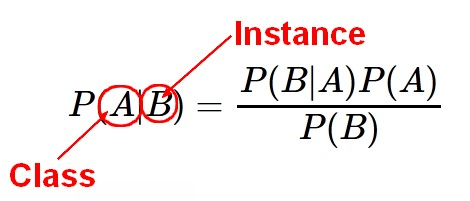# Data Mining - Generative Model

Generative model

Generative models are typically more flexible than discriminative models in expressing dependencies in complex learning tasks.

Generative model returns probabilities.

Generative model is a full probabilistic model of all variables, whereas a discriminative model provides a model only for the target variable(s) conditional on the observed variables. Thus a generative model can be used, for example, to simulate (i.e. generate) values of any variable in the model, whereas a discriminative model allows only sampling of the target variables conditional on the observed quantities.

## Model

Recommended PagesData Mining - (Discriminative|conditional) models

Discriminative models, also called conditional models, are a class of models used in machine learning for modeling the dependence of an unobserved variable y on an observed variable x. Discriminative...Data Mining - (Function|Model)

The model is the function, equation, algorithm that predicts an outcome value from one of several predictors. During the training process, the models are build. A model uses a logic and one of several...Data Mining - Naive Bayes (NB)

Naive Bayes (NB) is a simple supervised function and is special form of discriminant analysis. It's a generative model and therefore returns probabilities. It's the opposite classification strategy...